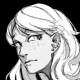# Geometry Clipmaps Revisited - Gamedev

2018.08.25 18:47:50
IndexA while back I wrote a bit about my adventures with a game engine technique called Geometry Clipmaps and my difficulties in implementing them. After spending what was supposed to be a week wherein I create a game fixing and completing an implementation of the Clipmaps instead, I think I'm now ready to talk about it. I'll also try to answer the questions I posed in the previous entry.

## Base Geometry

The clipmaps have a base resolution that defines the number of vertices used along an axis per level. We then construct a uniform grid of 1/4th of the vertices along each side. For instance, let's use a base resolution of 1024, so we construct a 256x256 grid. This grid is then used to form a 1024x1024 ring.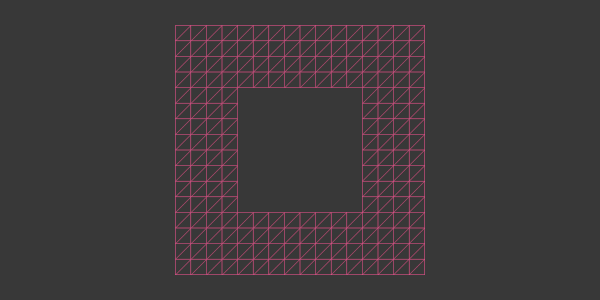The level-0 ring uses four more instances of this grid to fill out the middle, but every other ring uses the exact same geometry, just scaled up by a power of two.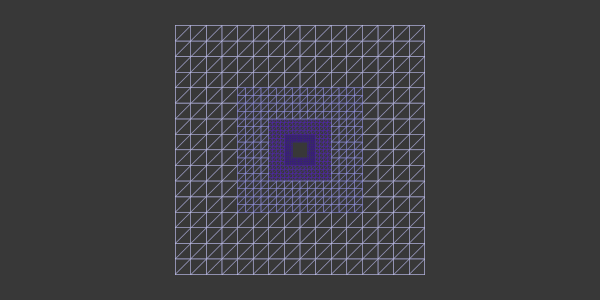This means we only need a single 256x256 vertex array to render everything. We can even reduce the number of draw calls to one by creating an additional vertex buffer that contains all the per-level grid offsets and level indices, and using instanced rendering. This additional buffer only requires `((12*l)+4)*3` elements, so with a typical 5 levels that would be a negligible 192 floats. This could be further reduced, but as it is it should be good enough for now.

## Map Representation

Since each level is represented by 1024x1024 vertices, we need `l` number of 1024x1024 textures to represent the map data. We can make use of 2D array textures for this. You'll likely want multiple textures to represent all of your data (one for elevation, one for material blends, etc).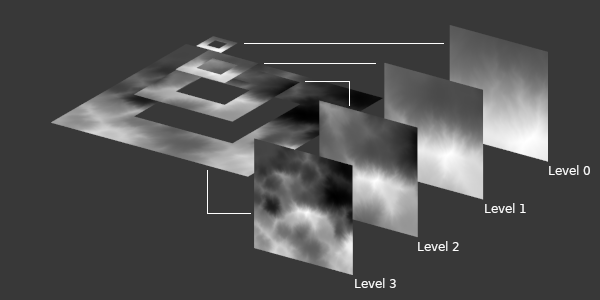In the vertex shader of the clipmap we then look up the value of the height texture corresponding to the current vertex position.

``````layout (location = 0) in vec3 position;
layout (location = 1) in float level;
layout (location = 2) in vec2 offset;

uniform mat4 view_matrix;
uniform mat4 projection_matrix;
uniform sampler2DArray height_map;

void main(){
float level_scale = pow(2.0, level);
float n = textureSize(height_map, 0).x;
vec2 map_pos = (position.xz + offset)/4;

vec2 tex_off = (map_pos+0.5-1/(n+1))*n;
float y = texelFetch(height_map, ivec3(tex_off, level), 0).r;

vec2 world_2d = map_pos * level_scale;
vec4 world = vec4(world_2d.x, y, world_2d.y, 1);
gl_Position =  projection_matrix * view_matrix * world;
}``````

Here, `position` is the position of the vertex in the grid, `level` is the current level index, and `offset` is the offset of the grid from the ring's centre. For simplicity's sake we assume the grid vertexes span between -0.5 and +0.5 and thus the offsets between -1.5 and +1.5.

Since the lookup of the texture is precise, we can use the pixel-aligned `texelFetch` function. You're also going to want a scaling factor in there somewhere in order to make sure you get the height you want, since it has been normalised into unit floats.

You can use the same fetch procedure for any additional maps you might have to retrieve their values and pass them on to the fragment shader, or whatever you like.

## Preparation Step

Once you export the full map data from your scene creator, you need to preprocess it into appropriate chunks before it can be used by the clipmaps. You need a directory of chunk files for each level of the clipmaps, with each chunk being of the clipmap resolution. So you start out with level 0, dice the map up and save the chunks to file. Then you subsequently scale the map down linearly by a factor of two and chunk it for the next level.

I recommend using a raw data format for the chunks that only contains the uncompressed pixel data without any header, so that loading it is efficient. Without compression or header you can simply mmap the file and pass the pointer to OpenGL to upload to textures.

## Update Step

Finally you need to implement an update step that takes care of uploading new map data to the texture arrays. This is the most complicated part of the whole technique and it has gone through several iterations as I've worked on it. The current version is almost perfect. There's a further optimisation that could be done, but I haven't felt the need to implement it yet.

In any case, since the clipmap chunks and the clipmap region coincide in size, any particular clipmap region can be covered by at most four chunks. So, in order to display a particular region, we upload the sub-regions of the four chunks that make up the region to each level of the clipmap array texture. We can do this thanks to `gl:pixel-store`'s `unpack-row-length`, which makes it possible to upload sub-regions of a source image.

Calculating the proper offsets isn't terribly exciting, but I've laid out the basic idea in the following image just for completeness' sake.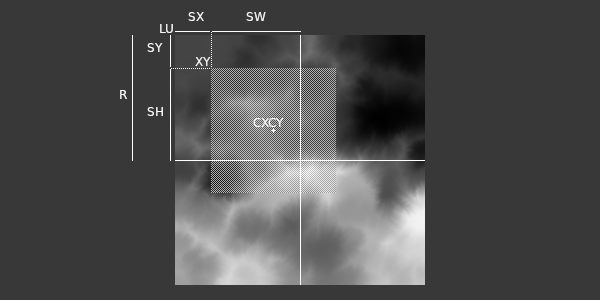And with this finally implemented, the clipmaps are complete!

Or so one would think. Unfortunately the devil is in the details, and there's a lot of those.

## Inter-Level Blending

Since each level above loses precision, the height values at the border between the levels is not going to match up exactly. In order to accommodate this, you have to blend smoothly between the two levels, gradually picking values from the higher level as you get closer to the edge.

At this point an attentive reader might notice that, since the vertex resolution is also not matching between two levels, the border is going to have a single edge of an outer level represented by two edges with a vertex in the middle of the inner level. Fortunately for us this is not an issue as long as we ensure that our clipmap textures use linear magnification interpolation. In that case, since the lines between vertices are linearly interpolated themselves, the lookup between two texels of the higher level is going to be interpolated in the same way, giving us a value that lands exactly on the middle point of the edge, just as we need it to be.

The blending factor itself can be calculated from the `map_pos` using some basic scaling and arithmetic.

``````vec2 alpha = (abs(map_pos)*2-0.5)*2;
alpha = clamp((alpha+BORDER_OFFSET-(1-BORDER_WIDTH))/BORDER_WIDTH, 0, 1);
float a = max(alpha.x, alpha.y);``````

The constants `BORDER_OFFSET` and `BORDER_WIDTH` allow you to easily designate how far from the border the blend should begin and how much you want to blend. I found `0.1` and `0.25` to be good values, though you might need to experiment depending on the resolution.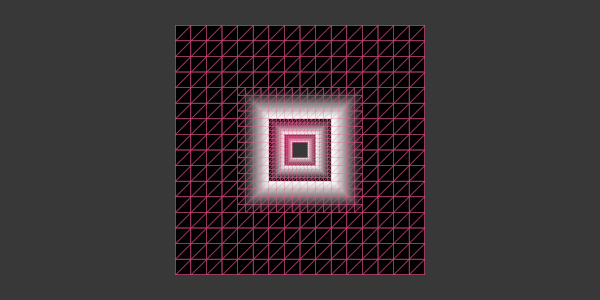At this point we simply perform another texture lookup in the outer level and mix the two together.

``````vec2 tex_off_o = (map_pos/2+0.5)-0.5/n;
float y_o = texture(height_map, vec3(tex_off_o, level+1)).r;
y = mix(y, y_o, a);``````

In this case we need to make use of the standard `texture` lookup, since we do want interpolation between texels. This also requires a slightly different texture coordinate calculation of course. You have to repeat this mixing procedure for every other map in order to smoothly transition between levels.

There's one more gotcha here, which is what happens at the outermost level. Since there's no higher level to blend with, we need to catch this. For this purpose we need a uniform that instructs us of the number of levels, and a check to see if we should blend or not.

``````layout (location = 0) in vec3 position;
layout (location = 1) in float level;
layout (location = 2) in vec2 offset;

uniform mat4 view_matrix;
uniform mat4 projection_matrix;
uniform sampler2DArray height_map;
uniform int levels;

void main(){
float level_scale = pow(2.0, level);
float n = textureSize(height_map, 0).x;
vec2 map_pos = (position.xz + offset)/4;

vec2 tex_off = (map_pos+0.5-1/(n+1))*n;
float y = texelFetch(height_map, ivec3(tex_off, level), 0).r;

if(level+1 < levels){
vec2 tex_off_o = (map_pos/2+0.5)-0.5/n;
float y_o = texture(height_map, vec3(tex_off_o, level+1)).r;
y = mix(y, y_o, a);
}

vec2 world_2d = map_pos * level_scale;
vec4 world = vec4(world_2d.x, y, world_2d.y, 1);
gl_Position =  projection_matrix * view_matrix * world;
}``````

With this in place we should now have a smooth display of the entire clipmap.

As long as we don't move, that is.

When the camera or player moves, the clipmaps need to be updated. We already have the update of the actual underlying elevation maps covered, but that's not good enough. Since the elevation maps are pixel-aligned, they are going to pop into place once the threshold has been crossed to load the next row of pixels in. Naturally this popping effect is very jarring and not acceptable.

Interestingly enough I don't recall any of the papers talking about this problem. I haven't re-read them to check, but I'm quite sure about it since I think I would have remembered.

In order to deal with this popping problem we have to physically move vertices by the remainder of the grid motion. As in, if our current level clipmap grid is unit-sized, then we need to shift the vertices in `xz` direction by `mod(pos, 1.0)` to compensate for the movement.

``````vec2 mov_off = mod(world_pos.xz, level_scale)/n;
vec2 world_2d = (map_pos * level_scale) - mov_off;``````

Naturally, we need to blend this between regions as well.

``````vec2 mov_off_o = mod(world_pos.xz, level_scale*2)/n;
mov_off = mix(mov_off, mov_off_o, a);``````

This will make the height transitions look buttery smooth, even in the face of continuous movement. It's almost perfect.

Since we shift the physical vertices for the elevation, we don't need to change how the values are looked up in the texture. This is not the same for any other map, which might be used for material lookup or other purposes in the fragment shader. The texture coordinates for those maps needs to be adjusted.

``````vec3 tex_i = vec3(tex_off/n, level);
vec3 tex_o = vec3(tex_off_o-a/(2*n), level+1);``````

This is almost perfect, but unfortunately there's still a small bit of popping going on, namely when two adjacent levels are off-by-one in their displayed maps. This effect is only really visible on low-resolution clipmaps, but I'd still like to figure out how to fix it at some point. At the time of writing this I had played around trying to fix it for around an hour and then put it off for later.

## Surface Normals

If you're going to use any kind of lighting engine, you're likely going to need surface normals for the clipmap. You could add another array texture that has this information baked in beforehand, but that would increase the amount of data that's being streamed quite a bit. It's easier and probably more efficient to just calculate the normals in the vertex shader.

``````float yu = texelFetch(height_map, ivec3(min(n-1, tex_off.x+1), tex_off.y, level), 0).r;
float yv = texelFetch(height_map, ivec3(tex_off.x, min(n-1, tex_off.y+1), level), 0).r;
vec3 normal = normalize(vec3(y-yu, 0.5, y-yv));``````

And of course we need to blend this as well.

``````float yu_o = texture(height_map, vec3(tex_off_o+vec2(1/n,0), level+1)).r;
float yv_o = texture(height_map, vec3(tex_off_o+vec2(0,1/n), level+1)).r;
yu = mix(yu, yu_o, a);
yv = mix(yv, yv_o, a);``````

## Concave Geometry

Since elevation maps can only represent convex geometry, concave geometry like caves, houses, and so forth need to be handled separately. In order to allow this, I attempted to use a simple geometry shader that punches holes into the terrain where the height value is zero.

``````layout(triangles) in;
layout(triangle_strip, max_vertices = 3) out;
in vec3 world[];

void main(){
if(world.y*world.y*world.y != 0){
int i;
for(i = 0;i < gl_in.length();i++){
gl_Position = gl_in[i].gl_Position;
EmitVertex();
}
EndPrimitive();
}
}``````

With the holes punched this would allow rendering in parts of the map that would be concave in place of the hole. Unfortunately it turns out that geometry shaders are super slow and this tears my frame rate in half. There's the other option of using `discard;` in the fragment shader, but that has its issues too. At this point I'm not sure yet how I'll handle this case.

Besides, I don't have a system for general mesh streaming yet, so I'll probably think about this once I get to it. If you have ideas though, please do let me know.

## Putting it All Together

Implementing this technique has been a long, arduous process. It has cost me multiple attempts and many hours of fiddling around and trying things out. Still, I'm quite happy to be able to say that I've got a technique implemented and working in Trial that is typically employed in big, commercial, triple-A titles.

You can find the current implementation of the clipmaps in the Trial repository. It's still making some shortcuts for me to test things, but I'm sure I'll get it to a usable, modular point soon enough. I hope that this entry has been helpful for people trying to understand this technique, and if not that, then I at least hope it has given people a bit of an appreciation for the work that has gone into it.

For now, here's a short in-engine rendering of a basic terrain region I generated using WorldCreator. It's rendered live using five levels of clipmaps with a base resolution of 1024x1024. Rendering is done using a very, very primitive phong renderer with solid colour mixing. No textures or anything fancy is applied, so it looks rather basic. The map is also not scaled quite right, so things look a bit squashed. My apologies for that.